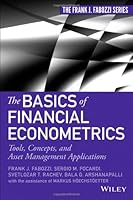# The Basics of Financial Econometrics## Book Description

An accessible guide to the growing field of financial econometrics

As and financial products have become more complex, financial econometrics has emerged as a fast-growing field and necessary foundation for anyone involved in quantitative . The techniques of financial econometrics facilitate the development and management of new financial instruments by providing models for pricing and risk assessment. In short, financial econometrics is an indispensable component to modern .

The Basics of Financial Econometrics covers the commonly used techniques in the field without using unnecessary mathematical/statistical analysis. It focuses on foundational ideas and how they are applied. Topics covered include: regression models, factor analysis, volatility estimations, and time series techniques. In addition, an associated website contains a number of real-world case studies related to important issues in this area.

• Covers the basics of financial econometrics—an important topic in quantitative finance
• Contains several chapters on topics typically not covered even in basic books on econometrics such as model selection, model risk, and mitigating model risk
• A companion website includes mini-cases that explain important topics in management, credit risk , option pricing, and

Geared towards both practitioners and finance students who need to understand this dynamic discipline, but may not have advanced mathematical training, this book is a valuable resource on a topic of growing importance.

Chapter 1 Introduction
Chapter 2 Simple Linear Regression
Chapter 3 Multiple Linear Regression
Chapter 4 Building And A Multiple Linear Regression Model
Chapter 5 Introduction To Time Series Analysis
Chapter 6 Regression Models With Categorical Variables
Chapter 7 Quantile Regressions
Chapter 8 Robust Regressions
Chapter 9 Autoregressive Moving Average Models
Chapter 10 Cointegration
Chapter 11 Autoregressive Heteroscedasticity Model And Its Variants
Chapter 12 Factor Analysis And Principal Components Analysis
Chapter 13 Model Estimation
Chapter 14 Model Selection
Chapter 15 Formulating And Implementing Investment Strategies Using Financial Econometrics

Appendix A Descriptive Statistics
Appendix B Continuous Probability Distributions Commonly Used In Financial Econometrics
Appendix C Inferential Statistics
Appendix D Of Matrix Algebra
Appendix E Model Selection Criterion: Aic And Bic
Appendix F Robust Statistics